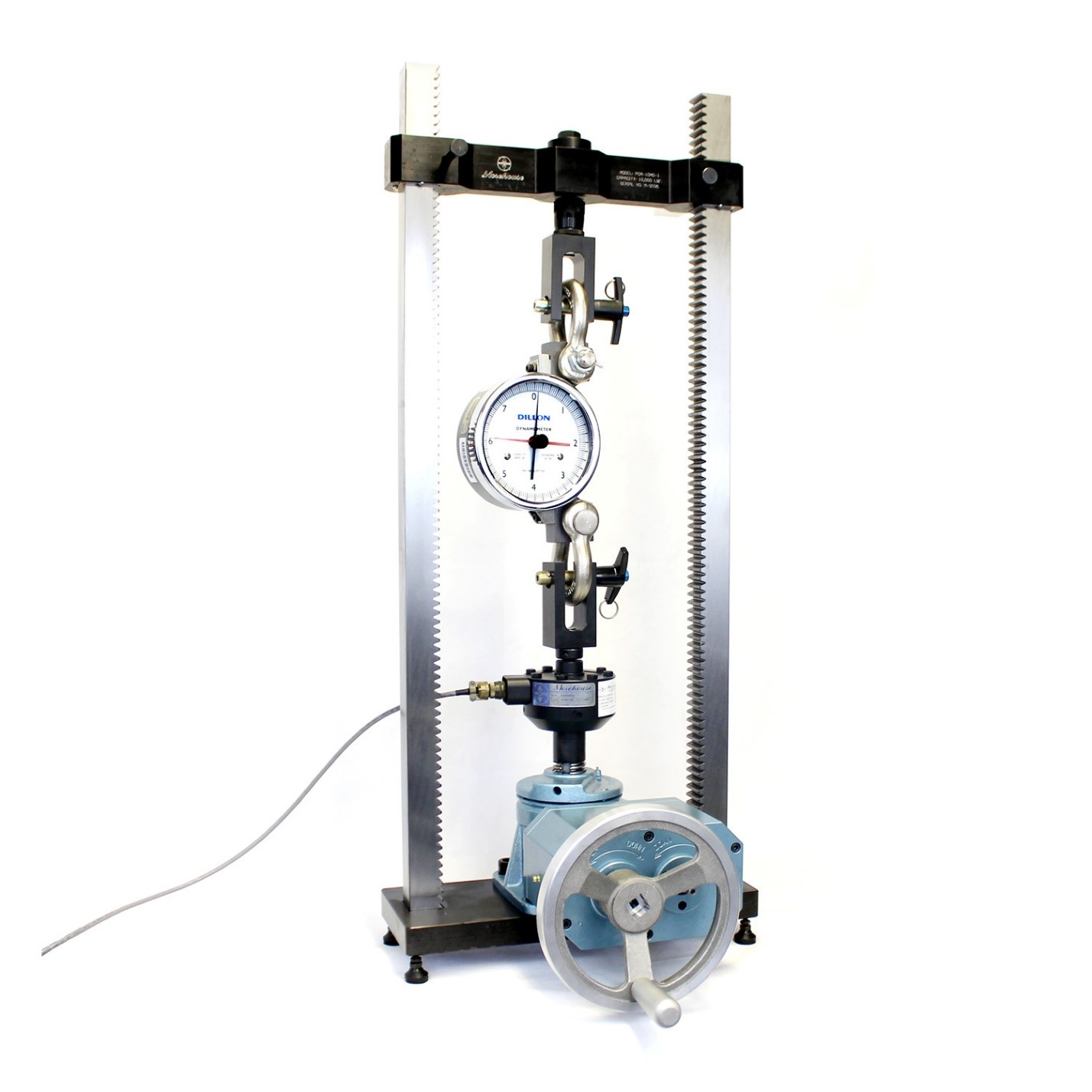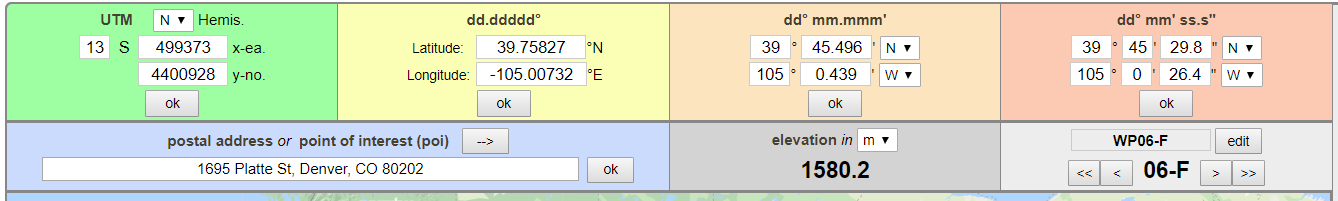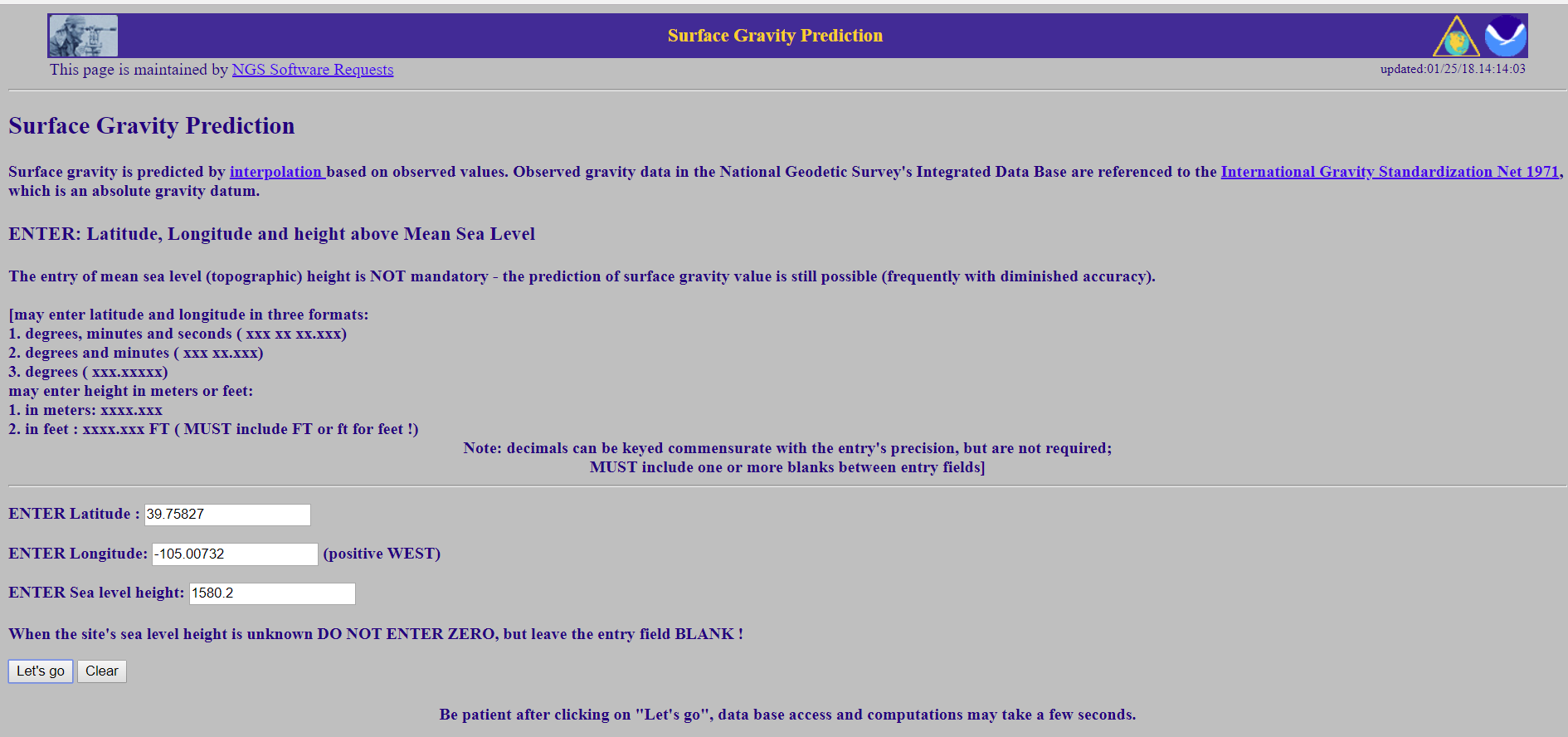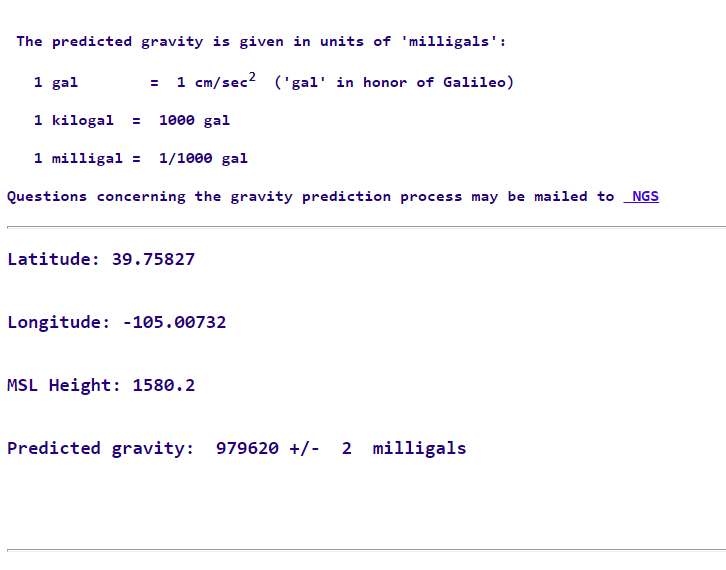Using Mass Weights to Calibrate Force Devices Can Result in a Large Measurement Error

When metrologists talk about measurement error, we’re talking about the difference between the nominal value and what the instrument is reading. If 10,000 lbf is applied to a force measuring device and the readout displays 10,002 lbf, then the device has a 2 lbf bias. If we load the same force measuring device to 10,002 lbf, we will have applied 10,000 lbf. This is a measurement error, and there can be many different causes. After speaking with several professionals inside the weighing industry, it has come to my attention that some labs use mass weights to calibrate force devices. These include dynamometers, crane scales, hand-held force gauges, and others. This can result in significant measurement error.

Let’s quickly review the difference between mass and force. Mass, under almost every terrestrial circumstance, is the measure of matter in an object. Measuring force takes additional factors into account: air density, material density, and gravity. It’s the effect of gravity which can produce significant errors when comparing mass and force measurements.

Gravity is not constant over the surface of the earth. The most extreme difference is 0.53% between the poles and the equator (983.2 cm/s2 at the former compared to 978.0 cm/s2 at the latter). A force measuring device calibrated in one location using mass weights then deployed somewhere else will produce different strains on the physical element. The resulting measurement errors can be significant.

Correcting for the difference in force and mass measurements is possible. When a device is adjusted for force measurements, the device will measure force without additional error for gravity correction, air density correction and so on needed. More information on converting force to mass and formulas can be found here.

Luckily, NOAA’s website has a tool for predicting local gravity anywhere on Earth. Here in York, Pennsylvania, Morehouse’s gravity is 9.801158 m/s2. If we compare that to the gravity of Houston, TX (9.79298 m/s2), we find the difference is -0.00084 ((9.79298 m/s2 - 9.801158 m/s2) / 9.79298 m/s2). As a percentage, that’s -0.084%.  If a lab in Houston calibrated a force measuring device with mass weights for use at Morehouse, we could expect anything we weigh to be heavy by 0.084%. This can have a lot of consequences. If we were shipping steel by the tonnage, we would ship less steel, reducing our cost but possibly upsetting our customer. Reversing the scenario, a scale calibrated in York with mass weights and used in Houston without correction, the steel supplier in Houston would ship more steel per ton.

As an aside, I should note that dynamometers, crane scales, tension links, handheld force gauges, and other similar devices are not always “Legal for Trade Scales”. Mainly, they can be used as force measuring devices because their displayed value can be adjusted based on a known force. If a known mass is used on-site, there is insignificant gravitational measurement error. The device can be used as a low-accuracy mass comparator. Since many of these instruments are used for measuring loads of 1 ton through 300 tons, it’s impractical to have the mass weights necessary to calibrate on-site and calibrating using force may be the only practical method to certify the device.

Another common example of these measurement errors occurs with scales (a mass measurement device). If 1,000 lbs mass is used to calibrate a scale at Morehouse and that scale is shipped to Denver, CO, it would have to be calibrated again or corrected by formula to obtain the proper mass. Just comparing the gravity in York (9.801158 m/s2 )and Denver (9.79620 m/s2 ), we find a difference of about 0.05%. Without correction, 1,000 lbs applied would read as 999.5 lbs. If the accuracy of the scale was 0.01%, then the device would be at least five times greater than the accuracy specification.

Morehouse manufactures force calibrating machines with varying degrees of mobility, including highly convenient 1-ton capacity Portable Calibrating Machines and our Benchtop Calibrating Machine (5-ton capacity, pictured top). These machines can be used either to calibrate in mass using a correction formula or in force. More Information on the benchtop calibrating machine can be found here.

Unless otherwise specified, Morehouse calibrates in pounds-force. To convert to mass measurements, we use a formula:

Force = M x g / 9.80665 m/s(1 – d/ D)

Where M = mass of weight, g = gravity at fixed location, d = air density, and D = material density

To convert to mass up to 120,000 lbf, the applied force is multiplied by 1.000712003. The difference in the percentage of using mass instead of force at Morehouse is 0.071 %.   The 1.000712003 includes corrections for air density as well as gravity.

For our application these values become ((mass * 9.801158 m/s2  )/9.80665 m/s2  ) * (1 – (0.001185/7.8334)

Force = mass x 0.999288781 or mass = Force x 1.000711725

At Morehouse, we’ve done calculations to determine our actual gravity and the results vary slightly from NOAA’s.

Morehouse Information from gravity determination on-site versus NOAA

Elevation: 126.2

From NOAA 9.80117 m/s

(From Morehouse determination on-site 9.801158 m/s)

Difference of 1.2 ppm or 0.00012 %

For capacities above 120,000 lbf, traceability will be derived from NIST deadweights, and we’ve applied different correction factors.

Below are some examples and steps to convert force to mass. We’re assuming Morehouse has performed the calibration in force units using deadweight primary standards accurate to 0.002% of applied force or better. If another lab performed the calibration using deadweight, then the value multiplied in Step 1 will be different.

Step 1. Convert Morehouse force values to Mass (Or if the lab used mass, then you could skip step 1)

Step 2. Find the gravity at the location of the measurement

Step 3. Find the ratio

Step 4. Multiply the Morehouse Mass value by the ratio

Example: In Denver, a brewery makes good beer partly because they take care when they weigh their ingredients. They want to use a crane scale to weigh in pounds. The crane scale was calibrated to 10,000 lbf at Morehouse. The brewery’s address is 1695 Platte St, Denver, CO 80202.

Step 1. Convert Morehouse force values to Mass

Multiply 10,000 by 1.000712003 = 10,007.12 lbs.

Step 2. Find the gravity at the location of the measurement

Use  https://www.geoplaner.com/ to get the Longitude and Latitude

Geoplaner shows

Latitude of 39.75827, Longitude of -105.00732and an elevation of 1580.2 m.Figure 1: Geoplaner website

Then click on  http://www.ngs.noaa.gov/TOOLS/Gravity/gravcon.htmlFigure 2: Entering info at ngs.noaa.gov

And enter the informationFigure 3: ngs.noaa predicted gravity

The results are a predicted gravity of 9.79620 m/s.

Step 3. Find the ratio

Difference = 9.79620/9.80117 = 0.99949

Gravity % Difference between Denver and York ((9.79620-9.80117)/9.79620) = -0.051 % Denver would need to have heavier weights to generate 10,000 lbf of force.

Step 4. Divide  the Morehouse Mass value by the ratio

10,007.12 / 0.99949 = 10,012.23 lbs. estimated mass value when converted from the force at Morehouse.

Note: The main problem with using this formula is not knowing the true air density and material density.  Using this method will get you close, but there will be large errors.

The Correct Method that should be used for all force to mass conversions.

Step 1. Obtain Measured Force Value

10,000 lbf

Step 2. Find the gravity at the location of the measurement

9.79620 m/s2  .

Step 3. Find Air Density and Material Density

For Denver, at around 24 degrees C Air Density may be estimated at 0.960 kg/m3 and Material density assuming Stainless Steel is 7916.453 kg /m3

Step 4. Use the following Formula

Mass  = Force x 9.80665/(local gravity*(1-d/D)

Mass = 10,000 lbf x 9.80665/(9.79620*(1-0.960/7916.453))

Mass = 10,011.89 lbs

Using a Load Cell calibrated in force units to apply mass

Step 1. Obtain Measured Force Value and convert to N

Force = 10,000 lbf = 44482.219 N

Step 2. Find the gravity at the location of the measurement

Local Gravity = 9.79620 m/s2

Step 3 Convert to the Proper Units

Mass = Force / Local Gravity

Mass = 44482.219 / 9.79620 = 4540.76 kg

Covert back to unit needed 4540.76 kg to lbs = 4540.76 x 2.20462 = 10,010.656 lbs

Note: This method completely ignores air density and material density which carries more error and risk.  It seems this is the method most scale calibrators use.

More Examples

Now let’s try using that same crane scale to measure 10,000 pounds across America and we are weighing in areas using the same air density and material density.

In Houston (where my aunt lives, and they make beer)

9.79298 / 9.801158 = 0.999165609 (difference is - 0.084%)

When a brewery in Houston compares our force calibration to their mass calibration, they should have about a - 0.084% difference. When the device has an accuracy spec of 0.1% of full scale, this error source is significant: almost 85% of the allowable error. (More about uncertainty budgets can be found here.)

In Santa Rosa, CA (where they make the best IPA’s, sorry Vermont)

9.80006 / 9.801158 = 0.999887972 (difference is - 0.011%)

When a brewery in Santa Rosa compares our force calibration to their mass calibration, they should have about a - 0.011% difference. If the device has an accuracy spec of 0.1% of full scale, the measurement error is massive: over 10% of the allowable error.

In Dunedin, FL (where they make fantastic beer)

9.79189 / 9.801158 = 0.999054397 (difference is - 0.095%)

When a brewery in Dunedin compares our force calibration to their mass calibration, they should have about a - 0.095% difference. When the device has an accuracy spec of 0.1 % of full scale, this error source is tremendous: almost 100% of the allowable error.

In Anchorage, AK (where they make really, superb beer)

9.81928 / 9.801158 = 1.001848965 (difference is 0.185%)

When a brewery in Anchorage compares our force calibration to their mass calibration, they should have about a 0.185% difference. When the device has an accuracy spec of 0.1% of full scale, this error source is almost double the allowable error.

Why use breweries? Because I like beer and the ingredients all need to be weighed. Fortunately, most microbreweries are using floor scales that are calibrated on-site and in place.  However, as production runs increase, they may switch to other methods to weigh such as flowscales and load cells. It is important that this equipment be calibrated properly. Using corrections that do not account for Air Density and Material Density carries more error and risk. The correction back to mass from force works well, but the correction from mass to mass may be problematic if calibration is done suspended in air. An accurate calibration will account for air density, material density, and temperature corrections. In mass to mass comparisons, these are sometimes not known or accounted for. For more information on conversions, please click here.

As always, please convert responsibly whether it is mass to mass or force to mass!

Everything we do, we believe in changing how people think about force and torque calibration. Morehouse believes in thinking differently about force and torque calibration and equipment. We challenge the "just calibrate it" mentality by educating our customers on what matters, what causes significant errors, and focus on reducing them. Morehouse makes our products simple to use and user-friendly. And we happen to make great force equipment and provide unparalleled calibration services.

Wanna do business with a company that focuses on what matters most? Email us at info@mhforce.com.

Written by Henry Zumbrun

www.mhforce.com